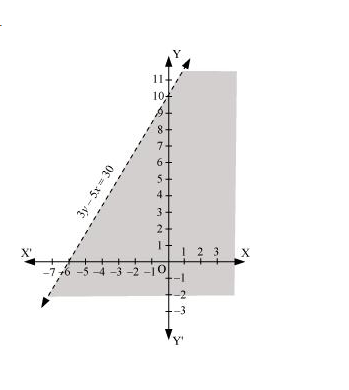# Solve the given inequality graphically in two-dimensional plane: 3y – 5x < 30Question:

Solve the given inequality graphically in two-dimensional plane: 3y – 5x < 30

Solution:

The graphical representation of 3y – 5x = 30 is given as dotted line in the figure below.

This line divides the xy-plane in two half planes.

Select a point (not on the line), which lies in one of the half planes, to determine whether the point satisfies the given inequality or not.

We select the point as (0, 0).

It is observed that,

3(0) – 5(0) < 30 or 0 < 30, which is true

Therefore, the upper half plane is not the solution region of the given inequality. Also, it is evident that any point on the line does not satisfy the given inequality.

Thus, the solution region of the given inequality is the half plane containing the point (0, 0) excluding the line.

The solution region is represented by the shaded region as follows.